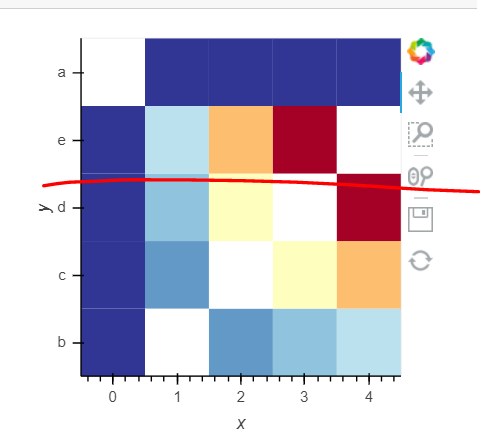# How to set categorical ylim

I know I can subset the data first, but is there a way to set ylim after the plot is generated, similar to how you can zoom in using the tools?

``````import holoviews as hv
data = [(i, chr(97+j),  i*j) for i in range(5) for j in range(5) if i!=j]
hm = hv.HeatMap(data).sort().opts(ylim=('a', 'e'))
hm
``````